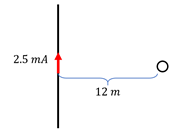# Problem: A circular coil with 200 loops and a radius of 0.15 mm is placed 12 m from a straight, current-carrying wire as shown in the following figure. What is the magnetic flux through the coil? Assume that the magnetic field is roughly constant through the coil, since 12 m &gt;&gt; 0.15 mm.

###### FREE Expert Solution
91% (340 ratings)
###### Problem Details

A circular coil with 200 loops and a radius of 0.15 mm is placed 12 m from a straight, current-carrying wire as shown in the following figure. What is the magnetic flux through the coil? Assume that the magnetic field is roughly constant through the coil, since 12 m >> 0.15 mm.Frequently Asked Questions

What scientific concept do you need to know in order to solve this problem?

Our tutors have indicated that to solve this problem you will need to apply the Magnetic Flux concept. You can view video lessons to learn Magnetic Flux. Or if you need more Magnetic Flux practice, you can also practice Magnetic Flux practice problems.

How long does this problem take to solve?

Our expert Physics tutor, Juan took 3 minutes and 24 seconds to solve this problem. You can follow their steps in the video explanation above.